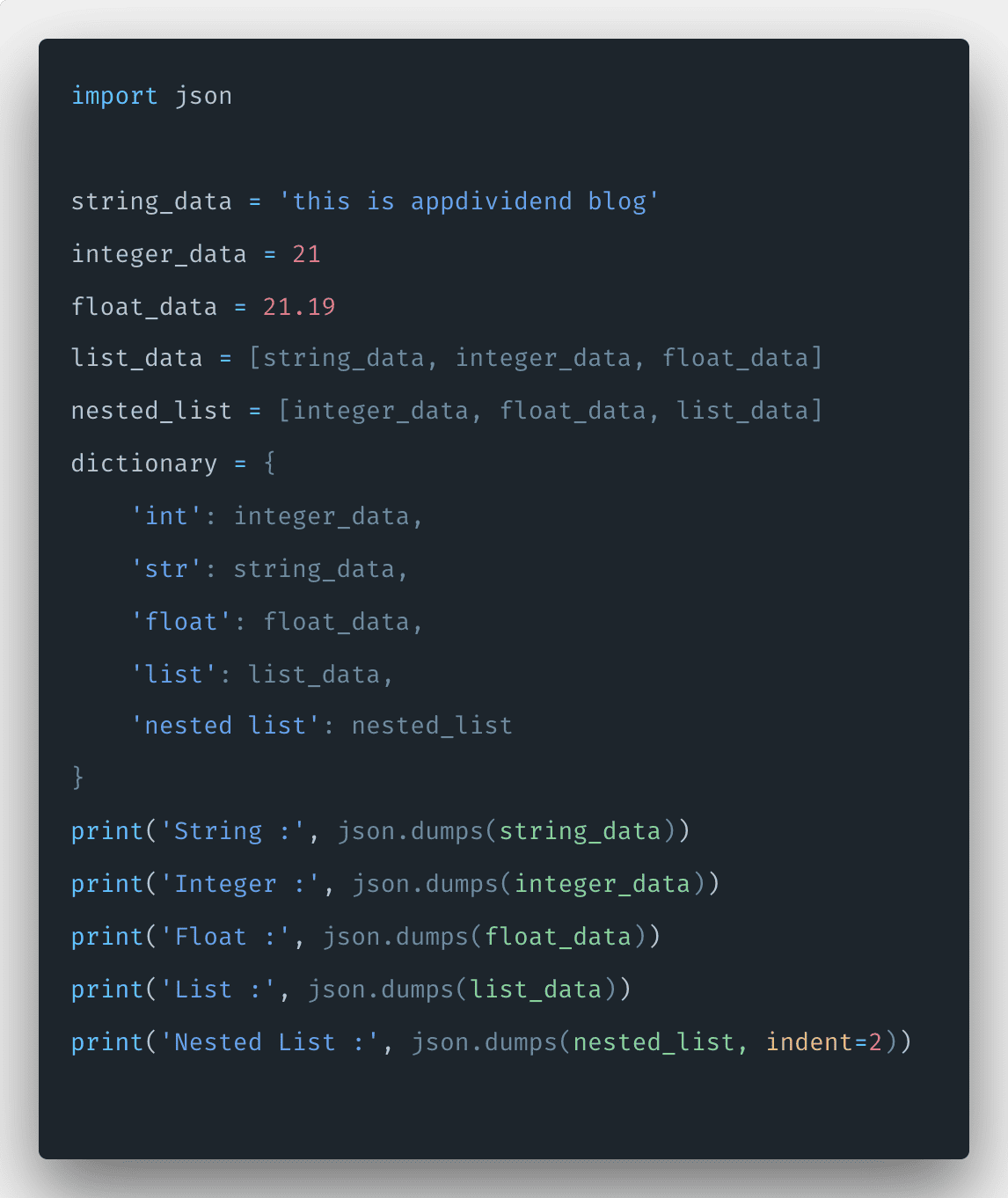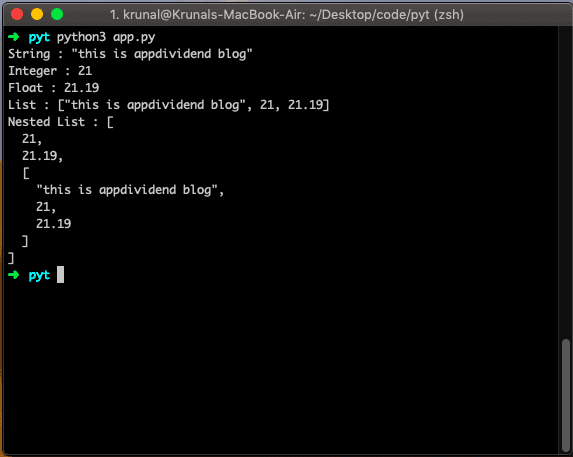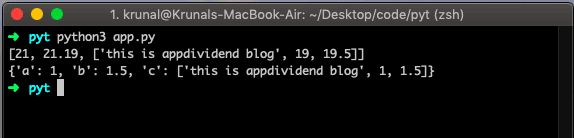# How to Parse JSON in Python

0
286JSON stands for JavaScript Object Notation, a text-based format that facilitates data interchange between diverse applications. For example, an application written in ASP.NET running on Windows Server can easily exchange JSON data with an application written in Python and running on Linux.

Python provides the json module, which can be imported to any file and used to parse JSON and generate JSON from python objects and lists.

The most common JSON entity you will encounter is an object: a set of key-value mappings in the format shown below.

```{
"firstName": "Krunal",
"lastName": "Lathiya",
"education": "IT Engineer",
"age": 25
}```

Here is how you can represent an array of objects.

In this representation, each item of the array is an object. JSON is a popular data format used for asynchronous browser/server communication. You can identify JSON using the following key points.

1. The data is only a name-value pair.
2. The comma separates data/Objects/arrays.
3. Curly braces hold an object.
4. Square holds the array.

## Python JSON.dumps()

The json.dumps() is a built-in Python json module method that converts an object into a json string. To convert any data to json format, use the json.dumps() method.

Let us take almost all types of data in the example, convert it into JSON, and print it in the console.

```# app.py

import json

string_data = 'this is appdividend blog'
integer_data = 21
float_data = 21.19
list_data = [string_data, integer_data, float_data]
nested_list = [integer_data, float_data, list_data]
dictionary = {
'int': integer_data,
'str': string_data,
'float': float_data,
'list': list_data,
'nested list': nested_list
}
print('String :', json.dumps(string_data))
print('Integer :', json.dumps(integer_data))
print('Float :', json.dumps(float_data))
print('List :', json.dumps(list_data))
print('Nested List :', json.dumps(nested_list, indent=2))```

See the output below.## How to parse json with Python

To parse json in Python, use the json.loads() method. The json.loads() is a built-in function that converts JSON data into Python data.

```# app.py

import json

arrayJson = '[21, 21.19, ["this is appdividend blog", 19, 19.5]]'
objectJson = '{"a":1, "b":1.5 , "c":["this is appdividend blog", 1, 1.5]}'

print(list_data)
print(dictionary_data)```

See the below output. We get the list and dictionary data.That’s it for this tutorial. Thanks for taking it.